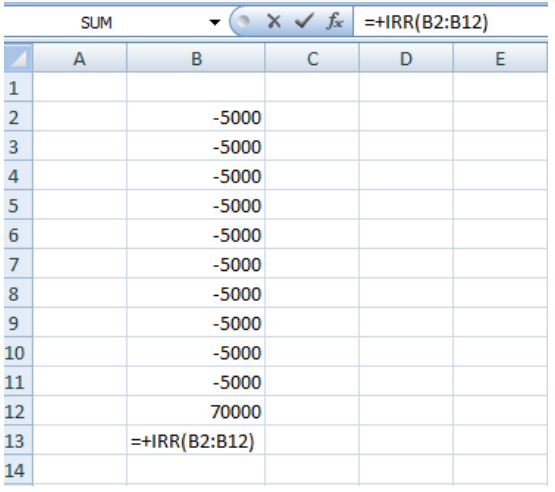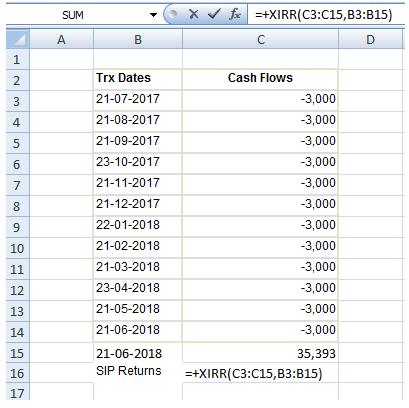# XIRR in Mutual Funds

The primary objective of any investment is to get returns. Returns can be in the form of income or capital appreciation or both. The two most popular measures for MF returns are compound annual growth rate (CAGR) and XIRR in mutual funds. CAGR, as the name suggests, is the rate at which your investment grows per annum over the investment period, assuming annual compounding. However, CAGR or point to point returns are not relevant or cannot be used in the case of multiple cash-flows, but XIRR in mutual funds can be used for multiple cash flows. XIRR meaning in mutual fund is to calculate returns on investments where there are multiple transactions taking place in different times. Full form of XIRR is Extended Internal Rate of Return.

## What are multiple cash-flows?

The simplest form of investment is one-time investment or lump sum in investment parlance and one-time redemption, i.e. selling your entire investment after a period of time. This involves two cash-flows, one cash-outflow (investment) and one cash-inflow (redemption). However, in many cases there can be multiple cash-inflows (e.g. SIP, additional purchases etc.) and multiple cash-outflows (e.g. SWP, dividends, partial redemptions etc.). CAGR cannot be used in such cases.

## Internal Rate of Return (IRR)

The metric used to measure the returns of a series of cash-flows is known as IRR or Internal Rate of Return. For the sake of simplicity, you can think of IRR as the annualized discounted cash-flows (DCF) rate of return. In this method, cash-flows are discounted at a certain rate (IRR) based on when the cash-flows happens to know the present value of investment (NPV). Cash-flows - it can either be inflows or outflows - which happen earlier in the investment tenure, are discounted less, while the ones which happen later are discounted more. This is due to time value of money, as value of money diminishes over time. IRR is the rate of discounting at which the present value (NPV) is zero.

Investors can use IRR to calculate the returns of their SIP, SWP, lump sum investment with additional purchases, multiple redemptions and other types of transactions involving multiple cash-flows. IRR takes into account all cash-flows – both, inflows and outflows - and the times at which cash-flows happen. The IRR equation is quite complex as one has to do trial and errors to calculate IRR manually. However, one can easily calculate IRR of cash-flows using the built in formula for IRR in Microsoft Excel Sheet as shown below -## Limitation of IRR

The biggest drawback of the IRR formula in excel sheet is that it assumes that the time interval between any consecutive cash-flows must be the same. This is a serious limitation, because time intervals between cash-flows happening are seldom the same throughout the tenure of the investment.

Let us take the example of a monthly SIP. Let us assume that the SIP date is on the 7th of every month. Even though it is a monthly SIP, the interval between two consecutive SIP instalments will vary from month to month because months have different number of days (28, 29, 30 or 31 days). Also, if your SIP date is on a holiday or falls on weekends in any month, the transaction will take place on the next business day, therefore the interval will be different. Similarly for dividend options, interval between dividend pay-outs may vary over the tenure of the investment. If the time intervals are not exactly the same, then excel sheet will return an incorrect value.

## Importance of XIRR in Mutual Funds

Fortunately, you can use a formula called XIRR in excel sheets which can take care of uneven cash-flow intervals. XIRR is simply a modification over IRR with the additional flexibility to assign specific dates to individual cash flows, thus making it a much more accurate calculation of returns. To know XIRR in mutual funds, all you have to do is to enter the transactions (SIP / SWP instalments, additional purchases, redemptions etc.) and the corresponding dates. You can get these transaction details from the statement of account sent by the fund house and apply the XIRR formula in excel sheet. Please see below for XIRR calculation of a simple SIP in excel sheet –## Points to be noted when calculating XIRR in Excel

To calculate XIRR in mutual funds, all cash-outflows (SIP instalments, lump sum purchases etc.) have to be entered as negative values (affix minus sign before the amount) and all cash-inflows (SWP, dividends, redemptions etc.) have to be entered as positive values. If you have not yet redeemed all your units then the current investment value needs to be entered along with the date of NAV, to calculate the XIRR of your MF investment. Some transactions like dividend re-investment do not involve actual cash-flows and therefore, should not be included in XIRR calculation. Switches are tricky, if you are calculating XIRR in MF at a scheme level, then switch should be treated as redemption i.e. cash out-flow or investment or the cash-inflow depending on whether you are calculating XIRR in mutual funds for source scheme (in the switch) or target scheme. However, if you are calculating XIRR in MF at a portfolio level then switch is irrelevant in XIRR calculation.

## Conclusion

In this article, we discussed meaning of XIRR in mutual fund and how it should be used to calculate multiple cash-flows e.g. SIP, SWP, additional purchase, dividends, partial redemptions etc. Many investors are familiar with CAGR. Like CAGR, XIRR in MF also uses the concept of compounding – in fact, you may think of XIRR as an aggregation of CAGRs. You must use XIRR if you have multiple cash-flows in your mutual fund investments.

An Investor Education and Awareness Initiative by Mirae Asset Mutual Fund.
For information on one-time KYC (Know Your Customer) process, Registered Mutual Funds and procedure to lodge a complaint in case of any grievance Click Here.

Mutual Fund investments are subject to market risks, read all scheme related documents carefully.

Close